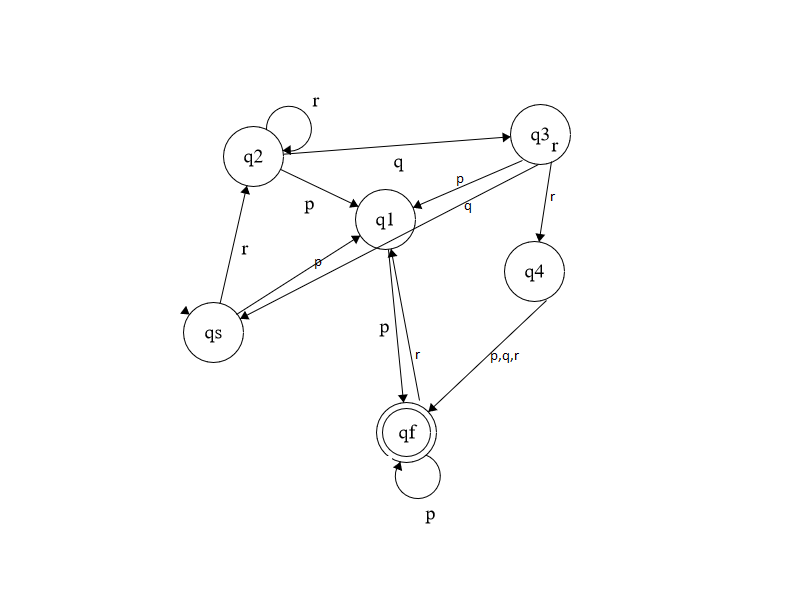# Question & Answer: Write a regular expression for the language……

(1)

Write a regular expression for the language.

Don't use plagiarized sources. Get Your Custom Essay on
Question & Answer: Write a regular expression for the language……
GET AN ESSAY WRITTEN FOR YOU FROM AS LOW AS \$13/PAGE

(2)

Define a finite state machine (FSM) that recognizes words in the language (input alphabet, states, start state, state transition table, and accept states). Include a state digraph for the FSM.

A: For alphabet {p,q,r}, all strings that contain the substring rqr or end with pp.

given alphabet{p,q,r}

a regular expression for DFA

now taking qf

putting the value of q4

qf=q1p+q3rp+q3rq+q4rr

now putting the value of q1

qf=qsp+q2p+q3p+q3rp+q3rq+q4rr

now putting the value of q3

qf= q2p+q2qp+q2qrp+q2qrq+q4qrr

qf= q2(p+qp+qrp+qrq+qrr)

regular expression for rqr will become

{pqr{r+q+r)*pqr}{(p+p)*}

transition table:state diagraph of the dfa: